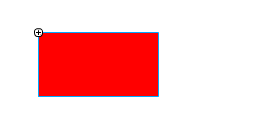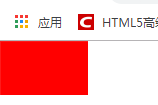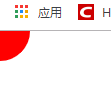## 新人必踩的shape坑

• 内容
• 评论
• 相关

```var _x = 100;
var _y = 200;
var shape = new createjs.Shape();
shape.graphics.beginFill("#ff0000").drawRect(_x,_y,300,200).endFill();
```var _x = 100;
var _y = 200;
var shape = new createjs.Shape();
shape.graphics.beginFill("#ff0000").drawRect(0,0,300,200).endFill();
shape.x = _x;
shape.y = _y;```1条评论